Homework Help Question & Answers

# Drag the account types to form the expanded accounting equation. Begin the equity section with capital....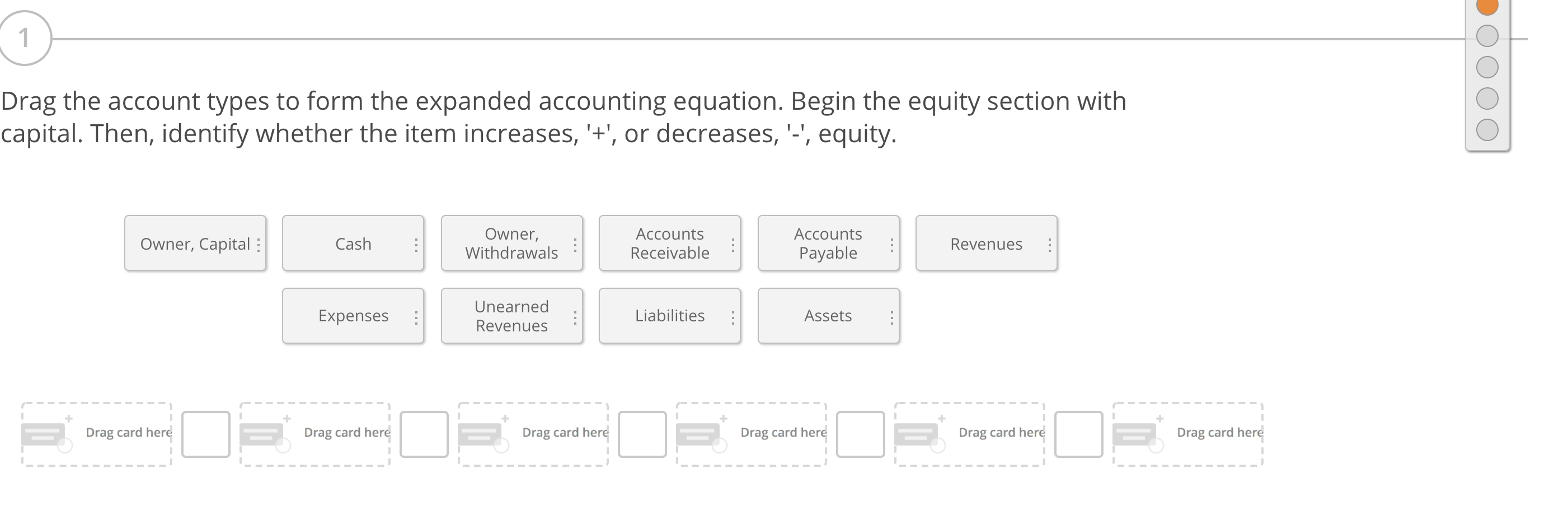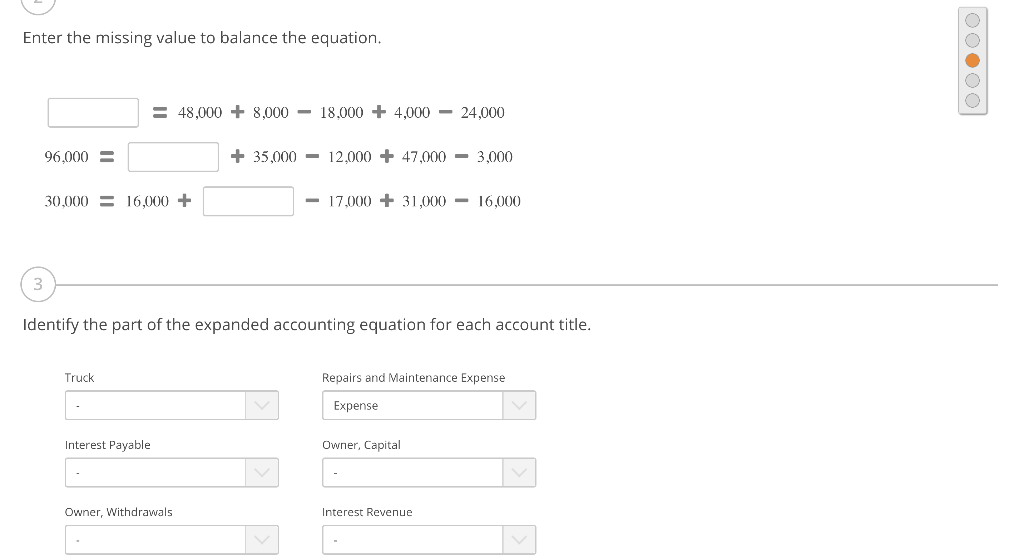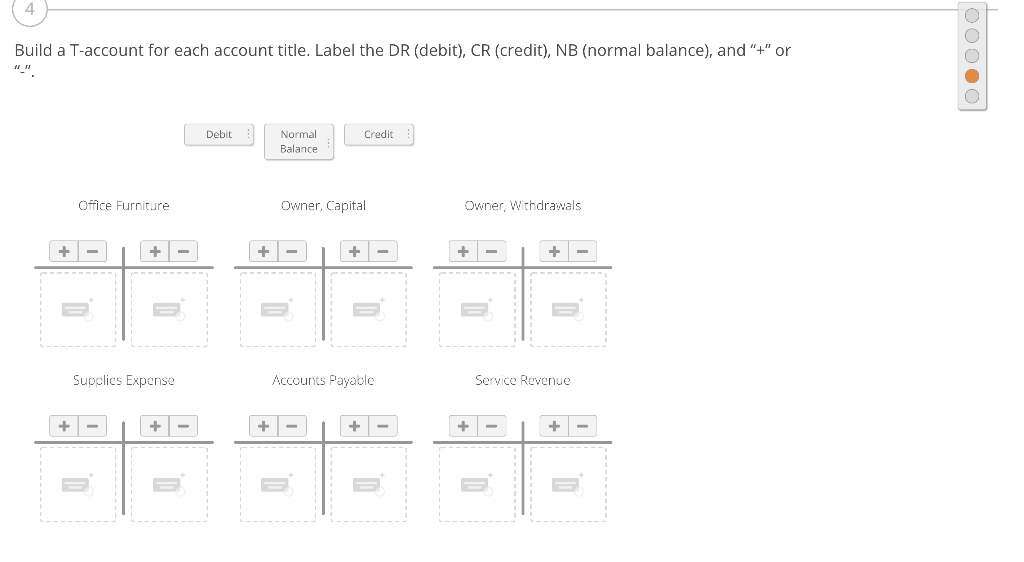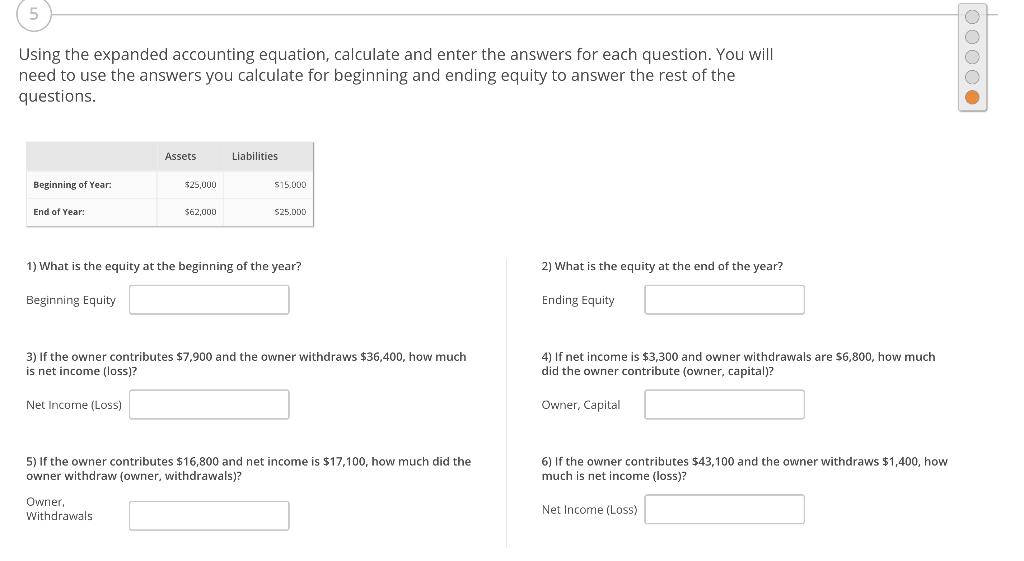Drag the account types to form the expanded accounting equation. Begin the equity section with capital. Then, identify whether the item increases, '+', or decreases, '', equity. Owner, Capital: Cash Owner, Withdrawals Accounts Receivable Accounts Payable Revenues Expenses Unearned Revenues Liabilities : Assets Drag card here Drag card here Drag card here Drag card here Drag card here Drag card here
Enter the missing value to balance the equation. = 48,000 + 8,000 – 18.000 + 4,000 - 24,000 96.000 = + 35,000 - 12.000 + 47,000 - 3,000 30,000 = 16,000 + - 17,000 + 31,000 - 16,000 3 Identify the part of the expanded accounting equation for each account title. Truck Repairs and Maintenance Expense Expense Interest Payable Owner, Capital Owner, Withdrawals Interest Revenue
Build a T-account for each account title. Label the DR (debit), CR (credit), NB (normal balance), and "+" or Debit Credit Normal Balance Office Furniture Owner, Capital Owner, Withdrawals 一一一一一一 Supplies Expense Accounts Payable Service Revenue + - +|-| + 十
Using the expanded accounting equation, calculate and enter the answers for each question. You will need to use the answers you calculate for beginning and ending equity to answer the rest of the questions. Assets Liabilities Beginning of Year: \$25,000 \$15.000 End of Year: \$62,000 S25.0DD 1) What is the equity at the beginning of the year? 2) What is the equity at the end of the year? Beginning Equity Ending Equity 3) If the owner contributes \$7,900 and the owner withdraws \$36,400, how much is net income (loss)? 4) If net income is \$3,300 and owner withdrawals are \$6,800, how much did the owner contribute (owner, capital)? Net Income (Loss) Owner, Capital 5) If the owner contributes \$16,800 and net income is \$17,100, how much did the owner withdraw (owner, withdrawals)? 6) If the ner contributes \$43,100 and the owner withdraws \$1,400, how much is net income (loss)? Owner Withdrawals Net Income (Loss)

#### Homework Answers

Answer #1

1) The accounting Equation is as follows.

Assets = Liabilities + Owners Equity + Revenues - Expenses - Owner's Withdrawal's

2)

18,000 = 48,000 + 8,000 - 18,000 + 4,000 - 24,000

96,000 = 29,000 + 35,000 - 12,000 + 47,000 - 3,000

30,000 = 16,000 + 16,000 - 17,000 + 31,000 - 16,000

3) Truck - Assets

Interest Payable - Liabilities

Owner Withdrawals - Owners withdrawals

Repairs and Maintenance Expense - Expense

Owners Capital - Owners Equity

Interest Revenue - Revenues

4)

 Office Furniture Owner Capitals Owner Withdrawal + - - + + - Debit Credit Debit Credit Debit Credit Normal Balance Normal Balance Normal Balance Supplies Expense Accounts Payable Service Revenue + - Debit Credit Debit Credit Debit Credit - + - + Normal Balance Normal Balance Normal Balance

5)

1) Beginning Equity = Beginning Assets - Beginning Liabilities

= \$ 25,000 - \$15,000

Beginning Equity = \$10,000

2)

Ending Equity = Ending Assets - Ending Liabilities

= \$ 62,000 - \$25,000

Ending Equity = \$37,000

3) Net Income ( Loss) = Beg Equity + Owner's Capital - Owner's Withdrawal - End Equity

= \$10,000 + \$7,900 - \$36,400 - \$37,000

Net Income ( Loss) = (\$55,500)

4) Owner Capital =  Net Income + Owner's Withdrawal + End Equity - Beg Equity

= \$3,300 + \$6,800 + \$37,000 - \$10,000

Owner Capital = \$37,100

5) Owner Withdrawals = Beg Equity +Owners Capital - Net Income - End Equity

= \$10,000 + \$16,800 + \$17,100 - \$37,000

Owner Withdrawals = \$6,900

6)

Net Income ( Loss) = Beg Equity + Owner's Capital - Owner's Withdrawal - End Equity

= \$10,000 + \$43,100 - \$1,400 - \$37,000

Net Income ( Loss) = \$14,700

Know the answer?
Your Answer:

#### Post as a guest

Your Name:

What's your source?

#### Earn Coin

Coins can be redeemed for fabulous gifts.

Not the answer you're looking for? Ask your own homework help question. Our experts will answer your question WITHIN MINUTES for Free.
Similar Homework Help Questions
• ### 1.2.3.4.5. Drag the account types to form the expanded accounting equation. Begin the equity section with...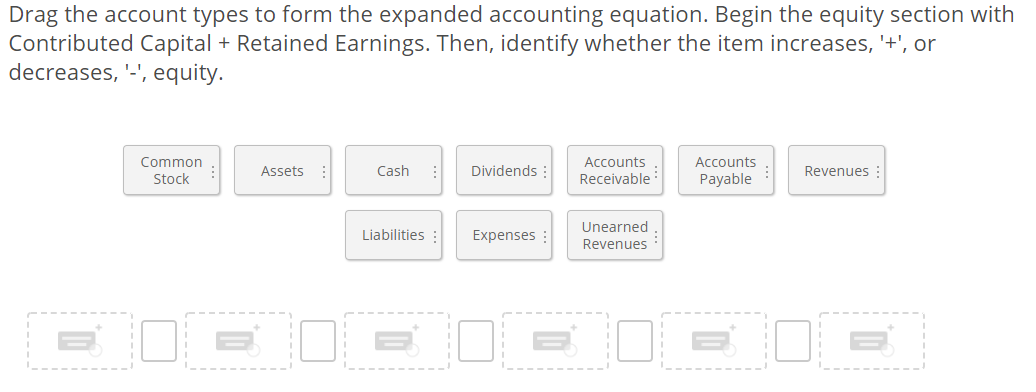1.2.3.4.5. Drag the account types to form the expanded accounting equation. Begin the equity section with Contributed Capital + Retained Earnings. Then, identify whether the item increases, '+', or decreases, '", equity. Common Stock : Assets : Cash : Dividends : Accounts Receivable Accounts Payable Revenues Liabilities: Expenses Unearned Revenues Enter the missing value to balance the equation. = 17,000 + 36,000 – 10,000 + 16,000 – 37,000 61,000 = + 40,000 – 32,000 + 10,000 – 2,000 68,000 =...

• ### Drag the account types to form the expanded accounting equation. Begin the equity section with Contributed...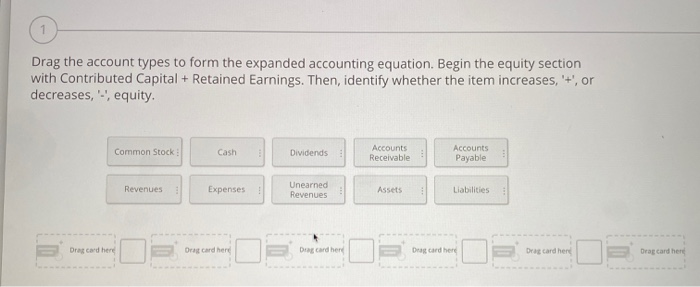Drag the account types to form the expanded accounting equation. Begin the equity section with Contributed Capital + Retained Earnings. Then, identify whether the item increases, '+', or decreases, equity. Common Stock Cash Dividends Accounts Receivable Accounts Payable Revenues Expenses Unearned Revenues Assets Liabilities Drag Card her Draard herd De card her Drag and her Drag card her Draard here

• ### drag the account type to form the expanded accounting equa Drag the account types to form...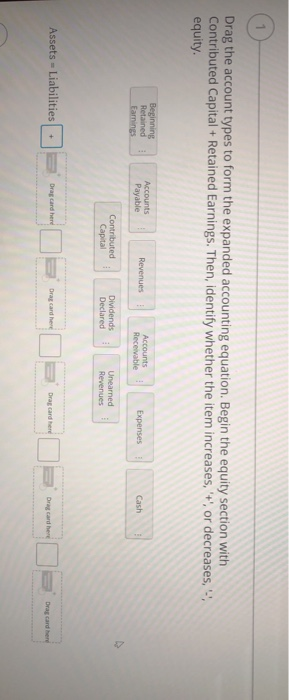drag the account type to form the expanded accounting equa Drag the account types to form the expanded accounting equation. Begin the equity section with Contributed Capital + Retained Earnings. Then, identify whether the item increases, +', or decreases, -', equity. Beginning Retained Accounts Payable Accounts Receivable Revenues Expenses Cash Earnings Contributed Capital Dividends Declared Unearned Revenues Assets = Liabilities Drag card herd Drag card here Drag card here Drag card here Drag card here

• ### Using the expanded accounting equation, calculate and enter the answers for each question. You will need...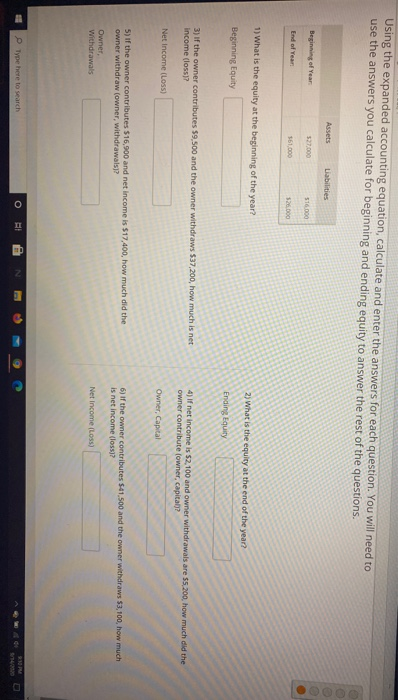Using the expanded accounting equation, calculate and enter the answers for each question. You will need to use the answers you calculate for beginning and ending equity to answer the rest of the questions. Assets Liabilities Beginning of Year \$27.000 516.000 End of Year 161.000 5260 1) What is the equity at the beginning of the year? 2) What is the equity at the end of the year? Beginning Equity Ending Equity 3) if the owner contributes 59 500 and...

• ### help pls! Using the expanded accounting equation, calculate and enter the answers for each question. You...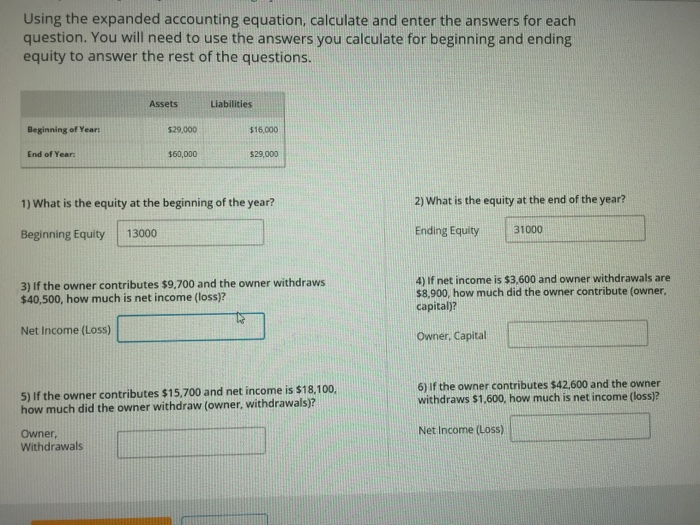help pls! Using the expanded accounting equation, calculate and enter the answers for each question. You will need to use the answers you calculate for beginning and ending equity to answer the rest of the questions. Assets Liabilities Beginning of Year: \$29.000 \$16.000 End of Year: \$60,000 \$29,000 2) What is the equity at the end of the year? 1) What is the equity at the beginning of the year? Beginning Equity 13000 Ending Equity 31000 3) If the owner...

• ### the expanded accounting equation, activity 3.a - classify the accounts The Expanded Accounting Equation Activity 3.a...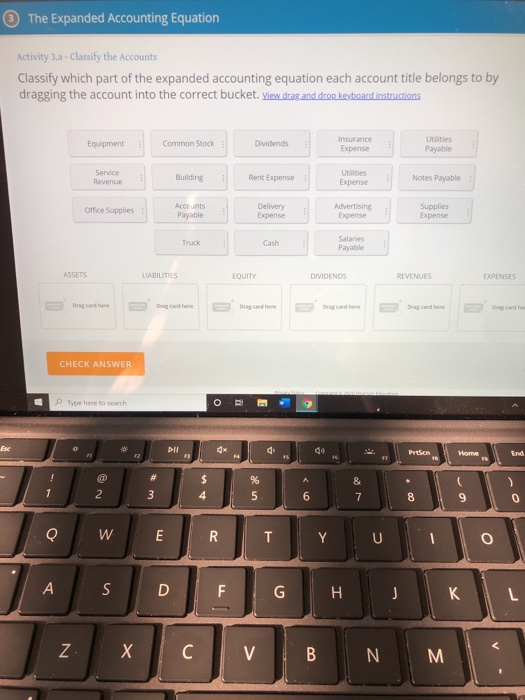the expanded accounting equation, activity 3.a - classify the accounts The Expanded Accounting Equation Activity 3.a - Classify the Accounts Classify which part of the expanded accounting equation each account title belongs to by dragging the account into the correct bucket. View drag and drop keyboard instructions Equipment Common Stock Dividends Insurance Expense Utilities Payable Service Revenue Building Rent Expense Utilities Expense Notes Payable Office Supplies Accounts Payable Delivery Expense Advertising Expense Supplies Expense Truck Cash Salaries Payable ASSETS LIABILITIES...

• ### help ! quesuull. You will need to use the answers you calculate for beginning and ending!...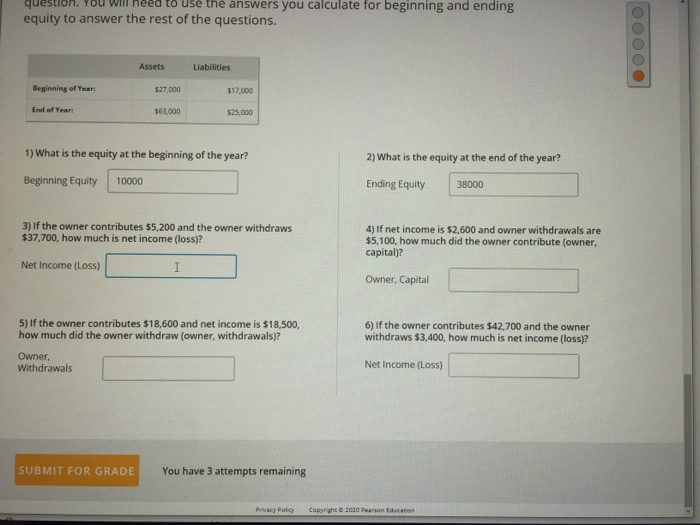help ! quesuull. You will need to use the answers you calculate for beginning and ending! equity to answer the rest of the questions. OOOOO Assets Liabilities Beginning of Years \$27.000 \$12.00 End of Year 161.000 \$25,000 1) What is the equity at the beginning of the year? 2) What is the equity at the end of the year? Beginning Equity 10000 Ending Equity 38000 3) If the owner contributes \$5,200 and the owner withdraws \$37,700, how much is net...

• ### The initial images are unreadable but i took pictures of the problems. Just skip the first...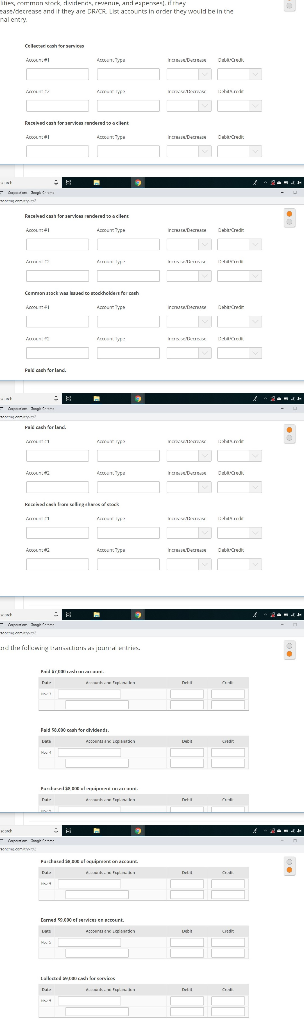The initial images are unreadable but i took pictures of the problems. Just skip the first few as I don’t know how to delete them. Appreciate any help! was decrease and they are CRICS. L u res in crder they would be in the C . Exhdcast . derdacht KOL:1 30. Drag the account types to form the expanded accounting equation. Begin the equity section with Contributed Capital + Retained Earnings. Then, identify whether the item increases,'+, or decreases,'", equity....

• ### Activity th - Using the expanded Accounting Equation Using the expanded accounting equation, calculate and enter...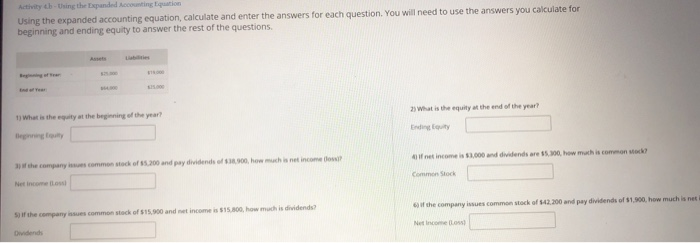Activity th - Using the expanded Accounting Equation Using the expanded accounting equation, calculate and enter the answers for each question. You will need to use the answers you calculate for beginning and ending equity to answer the rest of the questions 3) What is the equity at the end of the year? 1 What is the equity at the beginning of the year? Erity af net income is \$1,000 and dividends are 55,300,how much is hen the company common...

• ### What are the formulas to find the required information Using the expanded accounting equation, calculate and...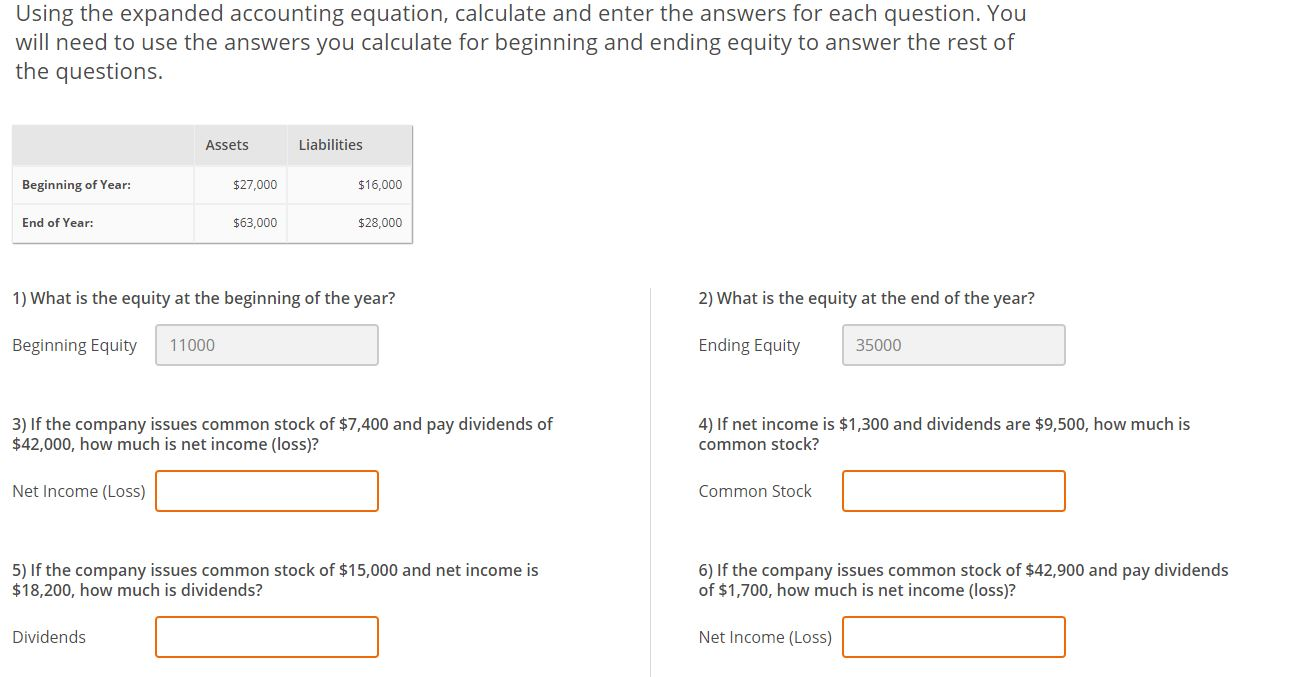What are the formulas to find the required information Using the expanded accounting equation, calculate and enter the answers for each question. You will need to use the answers you calculate for beginning and ending equity to answer the rest of the questions. Assets Liabilities Beginning of Year: \$27,000 \$16,000 End of Year: \$63,000 \$28,000 1) What is the equity at the beginning of the year? 2) What is the equity at the end of the year? Beginning Equity 11000...